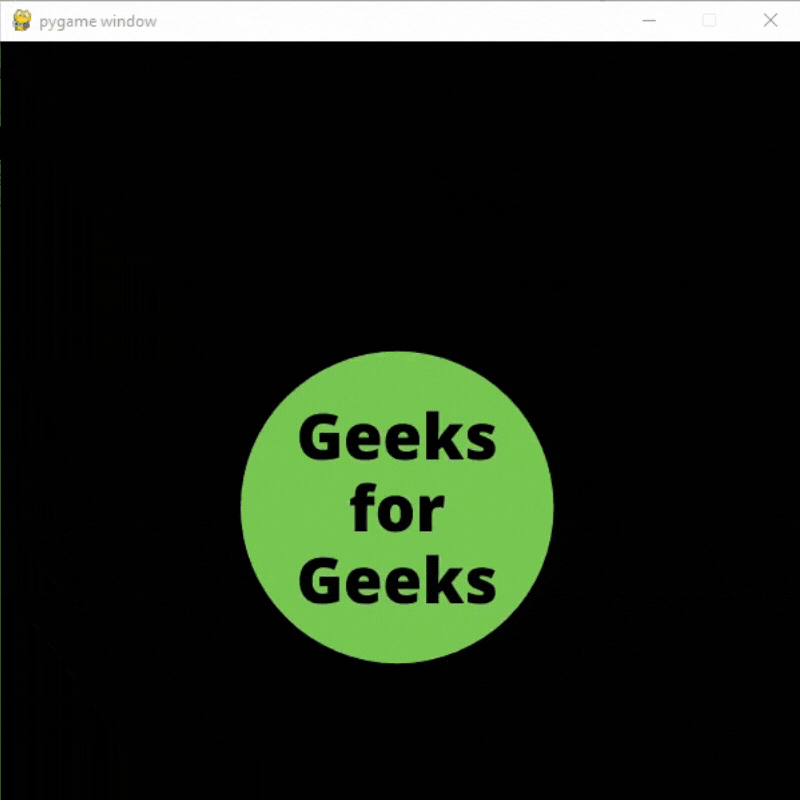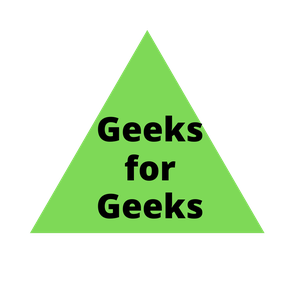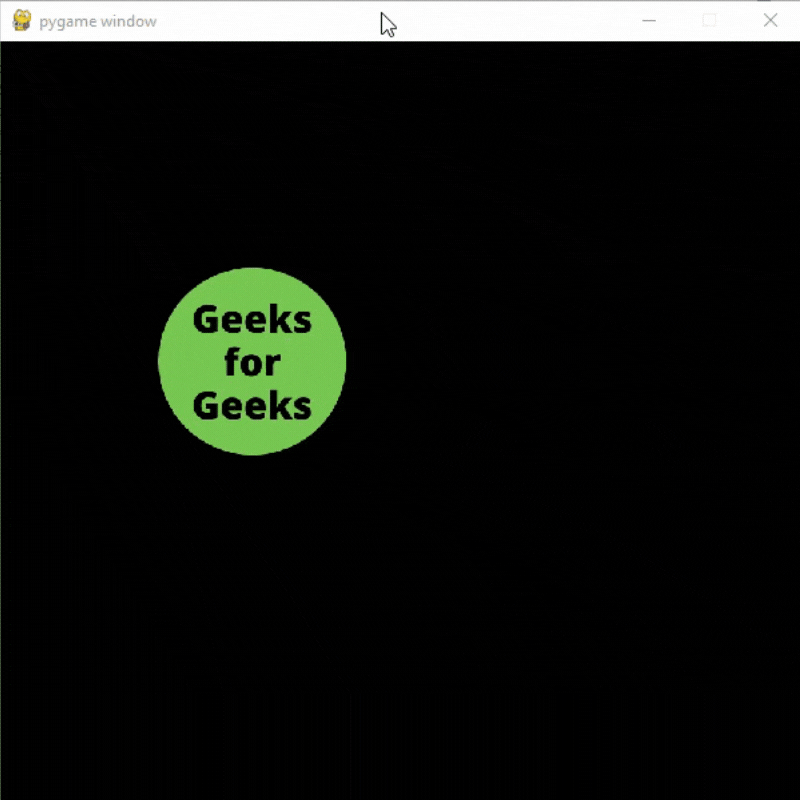# Pygame – Character Animation

• Difficulty Level : Expert
• Last Updated : 21 Sep, 2021

PyGame is a Free and Open source python library used to design video games. In this article, we will learn how we can add different animations to our characters.

## Simple Animation

We can easily add simple animations in our pygame projects by following the below steps.

Attention geek! Strengthen your foundations with the Python Programming Foundation Course and learn the basics.

To begin with, your interview preparations Enhance your Data Structures concepts with the Python DS Course. And to begin with your Machine Learning Journey, join the Machine Learning - Basic Level Course

• Create a list of sprites
• Iterate over the list
• Display the sprite on the screen## Python3

 `# Importing the pygame module``import` `pygame``from` `pygame.``locals` `import` `*` `# Initiate pygame and give permission``# to use pygame's functionality``pygame.init()` `# Create a display surface object``# of specific dimension``window ``=` `pygame.display.set_mode((``600``, ``600``))`  `# Create a list of different sprites``# that you want to use in the animation``image_sprite ``=` `[pygame.image.load(``"Sprite1.png"``),``                ``pygame.image.load(``"Sprite2.png"``)]`  `# Creating a new clock object to``# track the amount of time``clock ``=` `pygame.time.Clock()` `# Creating a new variable``# We will use this variable to``# iterate over the sprite list``value ``=` `0` `# Creating a boolean variable that``# we will use to run the while loop``run ``=` `True` `# Creating an infinite loop``# to run our game``while` `run:` `    ``# Setting the framerate to 3fps just``    ``# to see the result properly``    ``clock.tick(``3``)` `    ``# Setting 0 in value variable if its``    ``# value is greater than the length``    ``# of our sprite list``    ``if` `value >``=` `len``(image_sprite):``        ``value ``=` `0` `    ``# Storing the sprite image in an``    ``# image variable``    ``image ``=` `image_sprite[value]` `    ``# Creating a variable to store the starting``    ``# x and y coordinate``    ``x ``=` `150` `    ``# Changing the y coordinate``    ``# according the the value stored``    ``# in our value variable``    ``if` `value ``=``=` `0``:``        ``y ``=` `200``    ``else``:``        ``y ``=` `265` `    ``# Displaying the image in our game window``    ``window.blit(image, (x, y))` `    ``# Updating the display surface``    ``pygame.display.update()` `    ``# Filling the window with black color``    ``window.fill((``0``, ``0``, ``0``))` `    ``# Increasing the value of value variable by 1``    ``# after every iteration``    ``value ``+``=` `1`

Output:Explanation:

• Create a display surface object of a specific dimension
• Then Create a list of different sprites that you want to use in the animation, and then create a new clock object to track the amount of time.
• Create a boolean variable that we will use to run the while loop and then Create an infinite loop to run our game. Setting the framerate to 3fps just to see the result properly. Set 0 in value variable if its value is greater than the length of our sprite list.
• Store the sprite image in an image variable, and then Create a variable to store the starting x and y coordinate. Change the y-coordinate according to the value stored in our value variable.
• Then display the image in our game window and Update the display surface, and fill the window with black color.

## Moving Animation

If you only want to show animation when your character is moving or when a user presses a specific button then you can do that by following these steps:

• Create a variable to check if the character is moving or not.
• Create a list of sprites
• If the character is moving then iterate over the list of sprites and display the sprites on the screen.
• If the character is not moving display the sprite stored at the 0th index in the list.

#### Images Used:## Python3

 `# Importing the pygame module``import` `pygame``from` `pygame.``locals` `import` `*` `# Initiate pygame and give permission``# to use pygame's functionality``pygame.init()` `# Create a display surface object``# of specific dimension``window ``=` `pygame.display.set_mode((``600``, ``600``))`  `# Create a list of different sprites``# that you want to use in the animation``image_sprite ``=` `[pygame.image.load(``"Sprite1.png"``),``                ``pygame.image.load(``"Sprite2.png"``),``                ``pygame.image.load(``"Sprite3.png"``),``                ``pygame.image.load(``"Sprite4.png"``)]`  `# Creating a new clock object to``# track the amount of time``clock ``=` `pygame.time.Clock()` `# Creating a new variable``# We will use this variable to``# iterate over the sprite list``value ``=` `0` `# Creating a boolean variable that``# we will use to run the while loop``run ``=` `True` `# Creating a boolean variable to``# check if the character is moving``# or not``moving ``=` `False` `# Creating a variable to store``# the velocity``velocity ``=` `12` `# Starting coordinates of the sprite``x ``=` `100``y ``=` `150` `# Creating an infinite loop``# to run our game``while` `run:` `    ``# Setting the framerate to 10fps just``    ``# to see the result properly``    ``clock.tick(``10``)` `    ``# iterate over the list of Event objects``    ``# that was returned by pygame.event.get() method.``    ``for` `event ``in` `pygame.event.get():` `        ``# Closing the window and program if the``        ``# type of the event is QUIT``        ``if` `event.``type` `=``=` `pygame.QUIT:``            ``run ``=` `False``            ``pygame.quit()``            ``quit()` `        ``# Checking event key if the type``        ``# of the event is KEYUP i.e.``        ``# keyboard button is released``        ``if` `event.``type` `=``=` `pygame.KEYUP:` `            ``# Setting the value of moving to False``            ``# and the value f value variiable to 0``            ``# if the button released is``            ``# Left arrow key or right arrow key``            ``if` `event.key ``=``=` `pygame.K_LEFT ``or` `event.key ``=``=` `pygame.K_RIGHT:``                ``moving ``=` `False``                ``value ``=` `0` `    ``# Storing the key pressed in a``    ``# new variable using key.get_pressed()``    ``# method``    ``key_pressed_is ``=` `pygame.key.get_pressed()` `    ``# Changing the x coordinate``    ``# of the player and setting moving``    ``# variable to True``    ``if` `key_pressed_is[K_LEFT]:``        ``x ``-``=` `8``        ``moving ``=` `True``    ``if` `key_pressed_is[K_RIGHT]:``        ``x ``+``=` `8``        ``moving ``=` `True` `    ``# If moving variable is True``    ``# then increasing the value of``    ``# value variable by 1``    ``if` `moving:``        ``value ``+``=` `1` `    ``# Setting 0 in value variable if its``    ``# value is greater than the length``    ``# of our sprite list``    ``if` `value >``=` `len``(image_sprite):``        ``value ``=` `0` `    ``# Storing the sprite image in an``    ``# image variable``    ``image ``=` `image_sprite[value]` `    ``# Scaling the image``    ``image ``=` `pygame.transform.scale(image, (``180``, ``180``))` `    ``# Displaying the image in our game window``    ``window.blit(image, (x, y))` `    ``# Updating the display surface``    ``pygame.display.update()` `    ``# Filling the window with black color``    ``window.fill((``0``, ``0``, ``0``))`

Output:Explanation:

• Create a display surface object of a specific dimension and then create a list of different sprites that you want to use in the animation.
• Create a new clock object to track the amount of time and Create a new variable. We will use this variable to iterate over the sprite list. Create a boolean variable that we will use to run the while loop, and Create a boolean variable to check if the character is moving or not, create a variable to store the velocity starting coordinates of the sprite, and create an infinite loop to run our game and set the framerate to 10fps just to see the result properly, iterate over the list of Event objects that were returned by pygame.event.get() method. Close the window and program if the type of the event is QUIT.
• Checking event key if the type of the event is KEYUP i.e. keyboard button is released and set the value of moving to False and the value pf value variable to 0 if the button released is Left arrow key or right arrow key.
• Store the key pressed in a new variable using key.get_pressed() method. Change the x coordinate of the player and setting the moving variable to True. If the moving variable is True then increasing the value of the value variable by 1. Set 0 in the value variable if its value is greater than the length of our sprite list. Store the sprite image in an image variable and then Scale the image

My Personal Notes arrow_drop_up